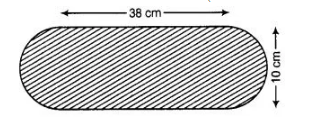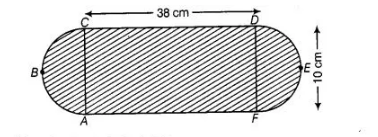# Find the area of the flower bed

Question:

Find the area of the flower bed (with semi-circular ends) shown in figureSolution:

Length and breadth of a circular bed are 38 cm and 10 cm.
∴                           Area of rectangle ACDF = Length x Breadth = 38 x 10 = 380 cm2Both ends of flower bed are semi-circles.

$\therefore \quad$ Radius of semi-circle $=\frac{D F}{2}=\frac{10}{2}=5 \mathrm{~cm}$

$\therefore \quad$ Area of one semi-circles $=\frac{\pi r^{2}}{2}=\frac{\pi}{2}(5)^{2}=\frac{25 \pi}{2} \mathrm{~cm}^{2}$

$\therefore \quad$ Area of two semi-circles $=2 \times \frac{25}{2} \pi=25 \pi \mathrm{cm}^{2}$

$\therefore \quad$ Total area of flower bed $=$ Area of rectangle $A C D F+$ Area of two semi-circles $=(380+25 \pi) \mathrm{cm}^{2}$